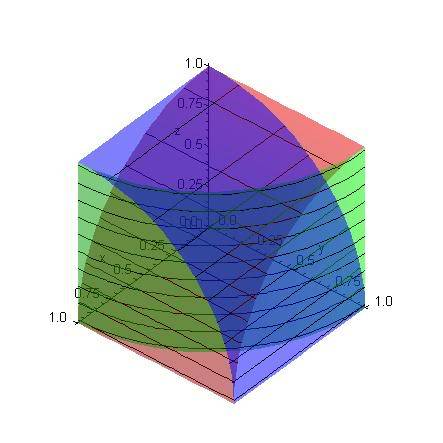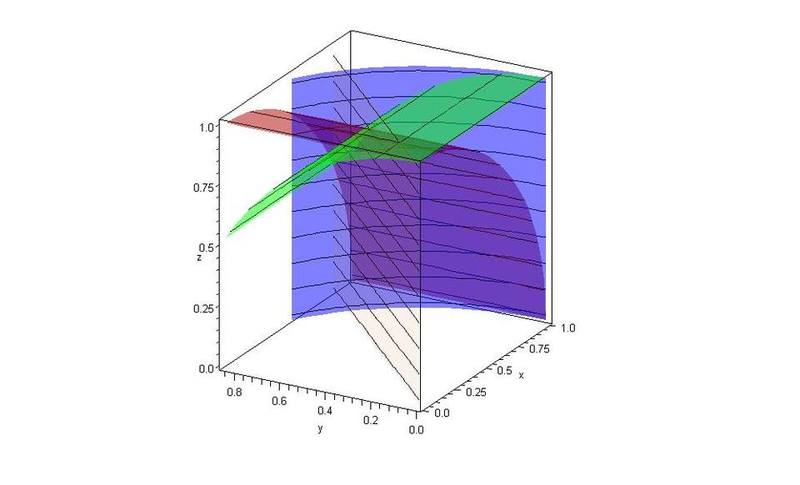# Volume of 3 intersecting Cylinders

• davidjoey

## Homework Statement

I am trying to find the volume of three intersecting cylinders that intersect at right angles given that the radius is 5.

I have found many sites that state just the answer, but I am after the proof for it showing all of the working. I know how to prove the rule for 2 intersecting cylinders, I can't do it for 3 though.

## Homework Equations

The rule I am after is 8(2-sqrt(2))r^3 or any similar form of that.

## The Attempt at a Solution

V_3(r,r,r) = 16r^3int_0^(pi/4)int_0^1ssqrt(1-s^2cos^2t)dsdt

A site i have found begins solving with that equation, but I have completely no idea where the numbers come from.The above is the looking-down view of the first octant. The next one is slightly cropped and from the z-axis looking out for getting the bounds of integration with the y=x plane included for reference.Try setting up the integral now... I'll use cylinders of radius R.

In cylindrical coordinates $$(r,\theta, z)$$: Using symmetry (multiply by 16), $$0\le \theta\le \scriptstyle{\frac{\pi}{4}}$$
here, z is bounded above by the red cylinder $$x^2+z^2=R^2$$ (second image) so $$0\le z\le \sqrt{R^2-r^2\cos^2\theta}$$
and r is bounded by the blue cylinder so $$0\le r\le R$$

so the integral is

$$16\int_{0}^{\scriptstyle{\frac{\pi}{4}}}\int_{0}^{R}\int_{0}^{\sqrt{R^2-r^2\cos^2\theta}}r\, dzdrd\theta = 16\int_{0}^{\scriptstyle{\frac{\pi}{4}}}\int_{0}^{R}r\sqrt{R^2-r^2\cos^2\theta}\, drd\theta$$
$$= -{\scriptstyle{\frac{16}{3}}} R^3\int_{0}^{\scriptstyle{\frac{\pi}{4}}} \frac{\sin^3\theta -1}{\cos^2\theta}\, d\theta = 8R^3\left( 2-\sqrt{2}\right)$$​

Last edited:
•